# Maharashtra Board Class 10 Maths-2 Solutions Chapter 1 Similarity Practice Set 1.2

## Maharashtra Board Class 10 Maths-2 Solutions Chapter 1 Similarity Practice Set 1.2

Question 1.
Given below are some triangles and lengths of line segments. Identify in which figures, ray PM is the bisector of ∠QPR.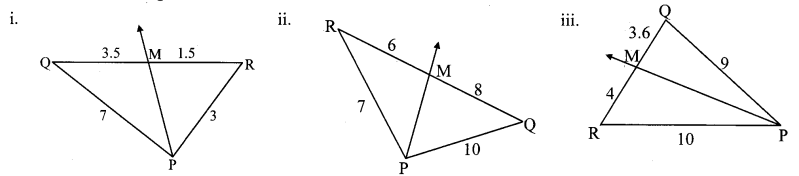Solution:
In ∆ PQR,
PQPR = 73 (i)
QMRM = 3.51.5 = 3515 = 73 (ii)
∴ PQPR = QMRM [From (i) and (ii)]
∴ Ray PM is the bisector of ∠QPR. [Converse of angle bisector theorem]

ii. In ∆PQR,
PQPR = 107 (i)
QMRM = 86 = 43 (ii)
∴ PQPR ≠ QMRM [From (i) and (ii)]
∴ Ray PM is not the bisector of ∠QPR

iii. In ∆PQR,
PQPR = 910 (i)
QMRM = 3.64 = 3640 = 910 (ii)
∴ PQPR = QMRM [From (i) and (ii)]
∴ Ray PM is the bisector of ∠QPR [Converse of angle bisector theorem]

Question 2.
In ∆PQR PM = 15, PQ = 25, PR = 20, NR = 8. State whether line NM is parallel to side RQ. Give reason.Solution:
PN + NR = PR [P – N – R]
∴ PN + 8 = 20
∴ PN = 20 – 8 = 12
Also, PM + MQ = PQ [P – M – Q]
∴ 15 + MQ = 25∴ line NM || side RQ [Converse of basic proportionality theorem]

Question 3.
In ∆MNP, NQ is a bisector of ∠N. If MN = 5, PN = 7, MQ = 2.5, then find QP.Solution:
In ∆MNP, NQ is the bisector of ∠N. [Given]
PNMN = QPMQ [Property of angle bisector of a triangle]
75 = QP2.5
∴ QP = 7×2.55
∴ QP = 3.5 units

Question 4.
Measures of some angles in the figure are given. Prove that APPB = AQQC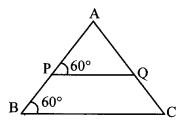Solution:
Proof
∠APQ = ∠ABC = 60° [Given]
∴ ∠APQ ≅ ∠ABC
∴ side PQ || side BC (i) [Corresponding angles test]
In ∆ABC,
sidePQ || sideBC [From (i)]
APPB = AQQC [Basic proportionality theorem]

Question 5.
In trapezium ABCD, side AB || side PQ || side DC, AP = 15, PD = 12, QC = 14, find BQ.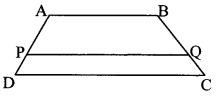Solution:
side AB || side PQ || side DC [Given]
APPD = BQQC [Property of three parallel lines and their transversals]
1512 = BQ14
∴ BQ = 15×1412
∴ BQ = 17.5 units

Question 6.
Find QP using given information in the figure.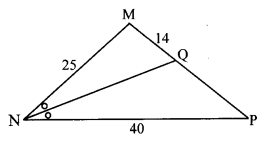Solution:
In ∆MNP, seg NQ bisects ∠N. [Given]
PNMN = QPMQ [Property of angle bisector of a triangle]
4025 = QP14
∴ QP = 40×1425
∴ QP = 22.4 units

Question 7.
In the adjoining figure, if AB || CD || FE, then find x and AE.Solution:
line AB || line CD || line FE [Given]
BDDF = ACCE [Property of three parallel lines and their transversals]
84 = 12X
∴ X = 12×48
∴ X = 6 units
Now, AE AC + CE [A – C – E]
= 12 + x
= 12 + 6
= 18 units
∴ x = 6 units and AE = 18 units

Question 8.
In ∆LMN, ray MT bisects ∠LMN. If LM = 6, MN = 10, TN = 8, then find LT.Solution:
In ∆LMN, ray MT bisects ∠LMN. [Given]
LMMN = LTTN [Property of angle bisector of a triangle]
610 = LT8
∴ LT = 6×810
∴ LT = 4.8 units

Question 9.
In ∆ABC,seg BD bisects ∠ABC. If AB = x,BC x+ 5, AD = x – 2, DC = x + 2, then find the value of x.Solution:
In ∆ABC, seg BD bisects ∠ABC. [Given]
ABBC = ADCD [Property of angle bisector of a triangle]
xx+5 = x2x+2
∴ x(x + 2) = (x – 2)(x + 5)
∴ x2 + 2x = x2 + 5x – 2x – 10
∴ 2x = 3x – 10
∴ 10 = 3x – 2x
∴ x = 10

Question 10.
In the adjoining figure, X is any point in the interior of triangle. Point X is joined to vertices of triangle. Seg PQ || seg DE, seg QR || seg EF. Fill in the blanks to prove that, seg PR || seg DF.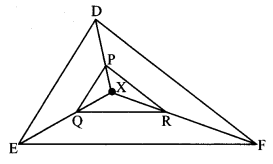Solution: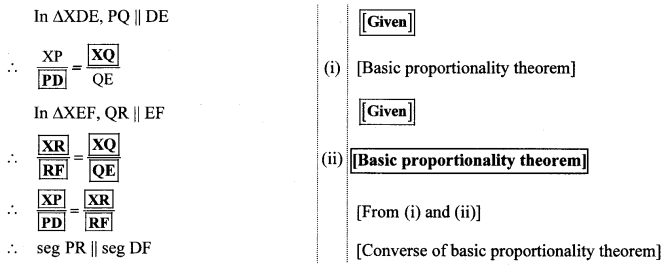Question 11.
In ∆ABC, ray BD bisects ∠ABC and ray CE bisects ∠ACB. If seg AB = seg AC, then prove that ED || BC.Solution:
In ∆ABC, ray BD bisects ∠ABC. [Given]
ABBC = AEEB (i) [Property of angle bisector of a triangle]
Also, in ∆ABC, ray CE bisects ∠ACB. [Given]
ACBC = AEEB (ii) [Property of angle bisector of a triangle]
But, seg AB = seg AC (iii) [Given]
ABBC = AEEB (iv) [From (ii) and (iii)]
ADDC = AEEB [From (i) and (iv)]
∴ ED || BC [Converse of basic proportionality theorem]

Question 1.
i. Draw a ∆ABC.
ii. Bisect ∠B and name the point of intersection of AC and the angle bisector as D.
iii. Measure the sides.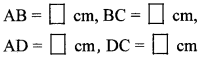iv. Find ratios ABBC and ADDC
v. You will find that both the ratios are almost equal.
vi. Bisect remaining angles of the triangle and find the ratios as above. Verify that the ratios are equal. (Textbook pg. no. 8)
Solution: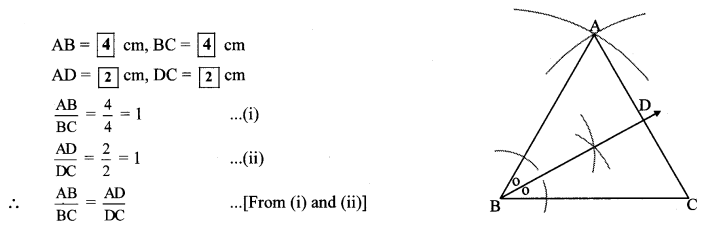Note: Students should bisect the remaining angles and verify that the ratios are equal.